# Making Decisions with the if Statement

## Making Decisions with the if Statement

The most common way to make a decision in Visual C++ is to use the if statement. You can use the if statement to perform a one-way test, a two-way test, a multiway test, or a nested test. Let’s consider a simple one-way test first.

### Performing One-Way Tests

The following illustration shows a simple one-way test.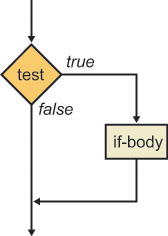The following example shows how to define a one-way test in Visual C++:

`if (number < 0) Console::WriteLine(S"The number is negative"); Console::WriteLine(S"The end");`

The if keyword is followed by a conditional expression enclosed in parentheses. (The parentheses are mandatory.) If the conditional expression evaluates to true, the next statement is executed—the message “The number is negative” will be displayed. Notice that the message “The end” will always be executed, regardless of the outcome of the test, because it is outside the if body.

 Note There is no semicolon after the closing parenthesis in the if test. One of the most common programming errors in C++ is to put one in by mistake, as shown here: `if (number < 0); // Note the spurious semicolon ` This statement is equivalent to the following statement, which is probably not what you intended: `if (number < 0) ; // Null if-body – do nothing if number < 0` If you want to include more than one statement in the if body, enclose the if body in braces ({}), as follows: `if (number < 0) { Console::Write(S"The number "); Console::Write(number); Console::WriteLine(S" is negative"); } Console::WriteLine(S"The end");`
 Tip It’s good practice to enclose the if body in braces, even if it comprises only a single statement. This style is defensive programming, in case you (or another developer) add more statements to the if body in the future.

In this exercise, you will create a new application to perform one-way tests. As this chapter progresses, you will extend the application to use more complex decision-making constructs and to perform loops.

For now, the application will ask the user to enter a date, and then it will perform simple validation and display the date in a user-friendly format on the console.

1. Start Microsoft Visual Studio .NET, and open a new Visual C++ Console Application (.NET) project. Name the application CalendarAssistant.

2. In Solution Explorer, double-click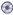CalendarAssistant.cpp to view the code file.

3. At the top of the file, immediately under the using namespace System; line, add the following function prototypes. (You will implement all these functions during this chapter.)

`int GetYear(); int GetMonth(); int GetDay(int year, int month); void DisplayDate(int year, int month, int day);`
4. At the end of the file, after the end of the _tmain function, implement the GetYear function as follows:

`int GetYear() { Console::Write(S"Year? "); String * input = Console::ReadLine(); int year = input->ToInt32(0); return year; }`
5. Implement the GetMonth function as shown in the following code. (This is a simplified implementation; later in this chapter, you will enhance the function to ensure that the user enters a valid month.)

`int GetMonth() { Console::Write(S"Month? "); String * input = Console::ReadLine(); int month = input->ToInt32(0); return month; }`
6. Implement the GetDay function as follows. (You will enhance this function later to ensure that the user enters a valid day in the given year and month.)

`int GetDay(int year, int month) { Console::Write(S"Day? "); String * input = Console::ReadLine(); int day = input->ToInt32(0); return day; }`
7. Implement the DisplayDate function as follows to display the date as three numbers. (Later in this chapter you will enhance this function to display the date in a more user-friendly format.)

`void DisplayDate(int year, int month, int day) { Console::WriteLine(S"\nThis is the date you entered:"); Console::Write(year); Console::Write(S"-"); Console::Write(month); Console::Write(S"-"); Console::Write(day); Console::WriteLine(); }`
8. Add the following code inside the _tmain method. This code asks the user to enter a year, month, and day. If the date passes a simplified validation test, the date is displayed on the console. If the date is invalid, it is not displayed at all.

`Console::WriteLine(S"Welcome to your calendar assistant"); Console::WriteLine(S"\nPlease enter a date"); int year = GetYear(); int month = GetMonth(); int day = GetDay(year, month); // Simplified test for now – assume there are 31 days in // every month :-) if (month >= 1 && month <= 12 && day >= 1 && day <= 31) { DisplayDate(year, month, day); } Console::WriteLine(S"\nThe end\n");`
 Note This if statement combines several tests by using the logical AND operator &&. As you learned in Chapter 3, logical tests are performed from left to right. Testing stops as soon as the final outcome is known for sure. For example, if the month is 0, there is no point performing the other tests—the date is definitely invalid. This is known as shortcut evaluation.
9. Build the program, and fix any compiler errors that you might have.

10. Run the program. Enter valid numbers for the year, month, and day (for example, 2001, 12, and 31). The program displays the messages shown in the following graphic.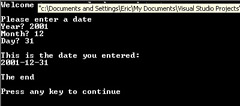Notice that the program displays the date because it is valid. The message “The end” is also displayed at the end of the program.

11. Run the program again, but this time, enter an invalid date (for example, 2001, 0, and 31). The program displays the messages shown in the following graphic.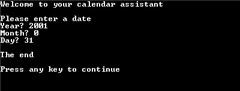Notice that the program doesn’t display the date because the date is invalid. Instead, the program just displays “The end” at the end of the program. You can make the program more user-friendly by displaying an error message if the date is invalid. To do so, use a two-way test.

### Performing Two-Way Tests

The following illustration shows a simple two-way test.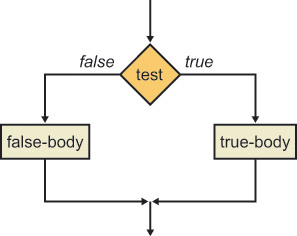The following code shows how to define a two-way test for the Calendar Assistant application:

`if (month >= 1 && month <= 12 && day >= 1 && day <= 31) { DisplayDate(year, month, day); } else { Console::WriteLine(S"Invalid date"); } Console::WriteLine(S"\nThe end\n");`

The else body defines what action to perform if the test condition fails.

In this exercise, you will enhance your Calendar Assistant application to display an error message if an invalid date is entered.

1. Continue working with the project from the previous exercise.

2. Modify the _tmain function so that it uses an if-else statement to test for valid or invalid dates.

`if (month >= 1 && month <= 12 && day >= 1 && day <= 31) { DisplayDate(year, month, day); } else { Console::WriteLine(S"Invalid date"); } Console::WriteLine(S"\nThe end\n");`
3. Build and run the program. Enter an invalid date, such as 2001, 0, and 31. The program now displays an error message.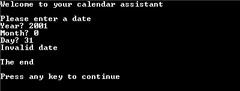### Performing Multiway Tests

You can arrange if-else statements in a cascading fashion to achieve multiway decision making. The following illustration shows multiway testing in a flowchart.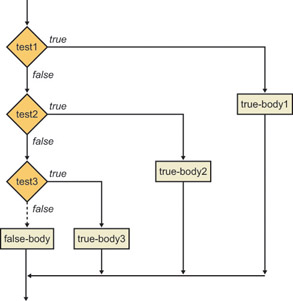The following code shows how to use a multiway test to determine the maximum number of days (maxDay) in a month:

`int maxDay; if (month == 4 || month == 6 || month == 9 || month == 11) { maxDay = 30; } else if (month == 2) { maxDay = 28; } else { maxDay = 31; }`

If the month is April, June, September, or November, maxDay is set to 30. If the month is February, maxDay is set to 28. (We’ll ignore leap years for now!) If the month is anything else, maxDay is set to 31.

 Note There is a space between the keywords else and if because they are distinct keywords. This is unlike Microsoft Visual Basic .NET, which has the single keyword ElseIf.

In this exercise, you will enhance your Calendar Assistant application to display the maximum number of days in the user’s chosen month.

1. Continue working with the project from the previous exercise.

2. Modify the GetDay function so that it uses an if-else-if statement to determine the maximum allowable number of days.

`int GetDay(int year, int month) { int maxDay; if (month == 4 || month == 6 || month == 9 || month == 11) { maxDay = 30; } else if (month == 2) { maxDay = 28; } else { maxDay = 31; } Console::Write(S"Day [1 to "); Console::Write(maxDay); Console::Write(S"]? "); String * input = Console::ReadLine(); int day = input->ToInt32(0); return day; } `

3. Build and run the program. Enter the year 2001 and the month 1. The program prompts you to enter a day between 1 and 31.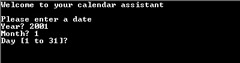Enter a valid day, and close the console window when the date is displayed.

4. Run the program again. Enter the year 2001 and the month 2. The program prompts you to enter a day between 1 and 28.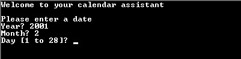Enter a valid day, and close the console window when the date is displayed. (Don’t worry about the date validation in _tmain. You will remove it later and replace it with more comprehensive validation in the GetMonth and GetDay functions.)

### Performing Nested Tests

You can perform nested tests, as shown in the following illustration.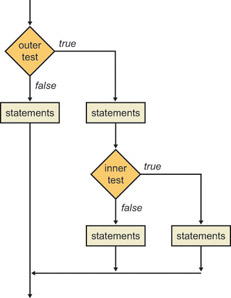The following code shows how to use nested tests to process leap years correctly in the Calendar Assistant application:

`int maxDay; if (month == 4 || month == 6 || month == 9 || month == 11) { maxDay = 30; } else if (month == 2) { bool isLeapYear = (year % 4 == 0 && year % 100 != 0) || (year % 400 == 0); if (isLeapYear) { maxDay = 29; } else { maxDay = 28; } } else { maxDay = 31; }`

If the month is February, we define a bool variable to determine if the year is a leap year. A year is a leap year if it is evenly divisible by 4 but not evenly divisible by 100 (except years that are evenly divisible by 400, which are leap years). The following table shows some examples of leap years and non-leap years.

Year

Leap Year?

1996

Yes

1997

No

1900

No

2000

Yes

We then use a nested if statement to test the bool variable isLeapYear so that we can assign an appropriate value to maxDay.

 Note There is no explicit test in the nested if statement. The condition if (isLeapYear) is equivalent to if (isLeapYear != false).

In this exercise, you will enhance your Calendar Assistant application to deal correctly with leap years.

1. Continue working with the project from the previous exercise.

2. Modify the GetDay function to match the block of code just described to test for leap years.

3. Build and run the program. Enter the year 1996 and the month 2. The program prompts you to enter a day between 1 and 29. Enter a valid day, and close the console window when the date is displayed.

4. Run the program again. Enter the year 1997 and the month 2. Verify that the program prompts you to enter a day between 1 and 28.

5. Run the program several more times using the test data from the previous table.Microsoft Visual C++ .NET(c) Step by Step
ISBN: 735615675
EAN: N/A
Year: 2003
Pages: 208

flylib.com © 2008-2017.
If you may any questions please contact us: flylib@qtcs.net